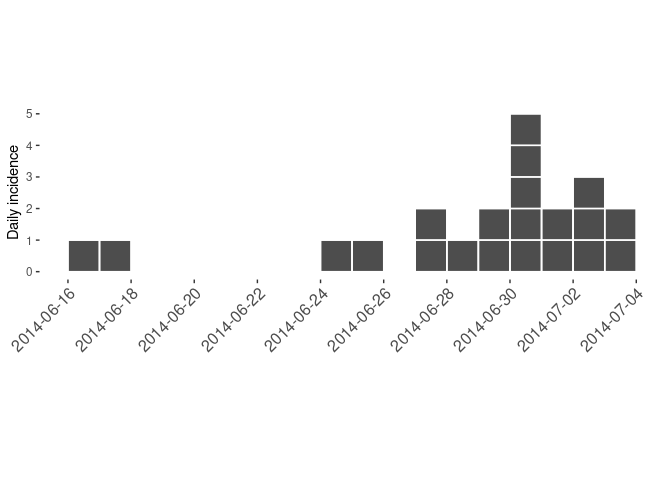# Customize plots of incidence

#### 2020-11-03

This vignette provides some tips for the most common customisations of graphics produced by plot.incidence. Our graphics use ggplot2, which is a distinct graphical system from base graphics. If you want advanced customisation of your incidence plots, we recommend following an introduction to ggplot2.

# Example data: simulated Ebola outbreak

This example uses the simulated Ebola Virus Disease (EVD) outbreak from the package outbreaks: ebola_sim_clean.

library(outbreaks)
library(ggplot2)
library(incidence)

onset <- ebola_sim_clean$linelist$date_of_onset
class(onset)
#>  "Date"
#>  "2014-04-07" "2014-04-15" "2014-04-21" "2014-04-27" "2014-04-26"
#>  "2014-04-25"

We compute the weekly incidence:

i <- incidence(onset, interval = 7)
i
#> <incidence object>
#> [5829 cases from days 2014-04-07 to 2015-04-27]
#> [5829 cases from ISO weeks 2014-W15 to 2015-W18]
#>
#> $counts: matrix with 56 rows and 1 columns #>$n: 5829 cases in total
#> $dates: 56 dates marking the left-side of bins #>$interval: 7 days
#> $timespan: 386 days #>$cumulative: FALSE

i.sex <- incidence(onset, interval = 7, group =  ebola_sim_clean$linelist$gender)
i.sex
#> <incidence object>
#> [5829 cases from days 2014-04-07 to 2015-04-27]
#> [5829 cases from ISO weeks 2014-W15 to 2015-W18]
#> [2 groups: f, m]
#>
#> $counts: matrix with 56 rows and 2 columns #>$n: 5829 cases in total
#> $dates: 56 dates marking the left-side of bins #>$interval: 7 days
#> $timespan: 386 days #>$cumulative: FALSE

i.hosp <- incidence(onset, interval = 7, group =  ebola_sim_clean$linelist$hospital)
i.hosp
#> <incidence object>
#> [5829 cases from days 2014-04-07 to 2015-04-27]
#> [5829 cases from ISO weeks 2014-W15 to 2015-W18]
#> [6 groups: Connaught Hospital, Military Hospital, other, Princess Christian Maternity Hospital (PCMH), Rokupa Hospital, NA]
#>
#> $counts: matrix with 56 rows and 6 columns #>$n: 5829 cases in total
#> $dates: 56 dates marking the left-side of bins #>$interval: 7 days
#> $timespan: 386 days #>$cumulative: FALSE

# The plot.incidence function

When calling plot on an incidence object, the function plot.incidence is implicitly used. To access its documentation, use ?plot.incidence. In this section, we illustrate existing customisations.

## Default behaviour

By default, the function uses grey for single time series, and colors from the color palette incidence_pal1 when incidence is computed by groups:

plot(i)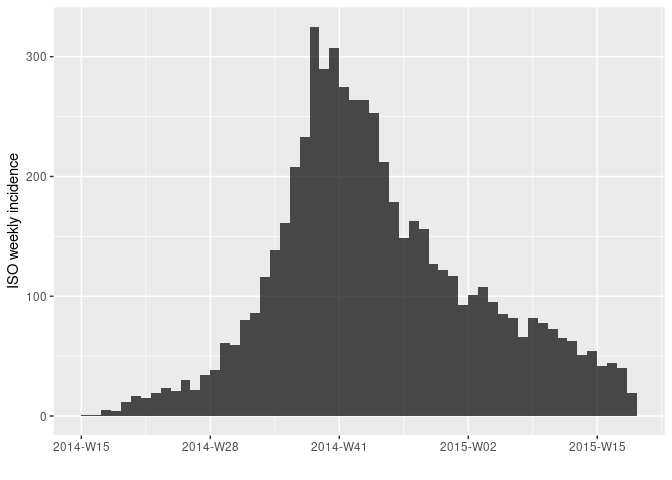plot(i.sex)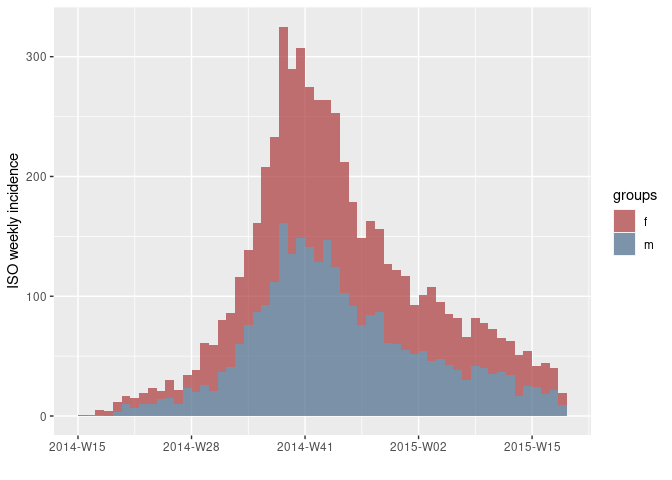plot(i.hosp)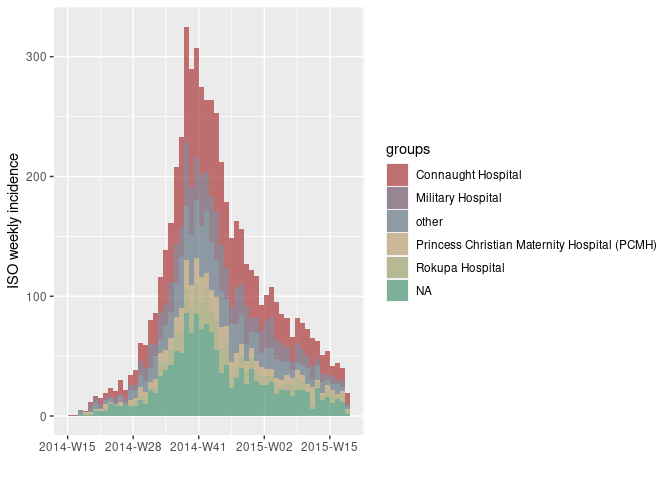However, some of these defaults can be altered through the various arguments of the function:

args(incidence:::plot.incidence)
#> function (x, ..., fit = NULL, stack = is.null(fit), color = "black",
#>     border = NA, col_pal = incidence_pal1, alpha = 0.7, xlab = "",
#>     ylab = NULL, labels_week = !is.null(x$weeks), labels_iso = !is.null(x$isoweeks),
#>     show_cases = FALSE, n_breaks = 6)
#> NULL

## Changing colors

### The default palette

A color palette is a function which outputs a specified number of colors. By default, the color used in incidence is called incidence_pal1. Its behaviour is different from usual palettes, in the sense that the first 4 colours are not interpolated:

par(mfrow = c(3, 1), mar = c(4,2,1,1))
barplot(1:2, col = incidence_pal1(2))
barplot(1:4, col = incidence_pal1(4))
barplot(1:20, col = incidence_pal1(20))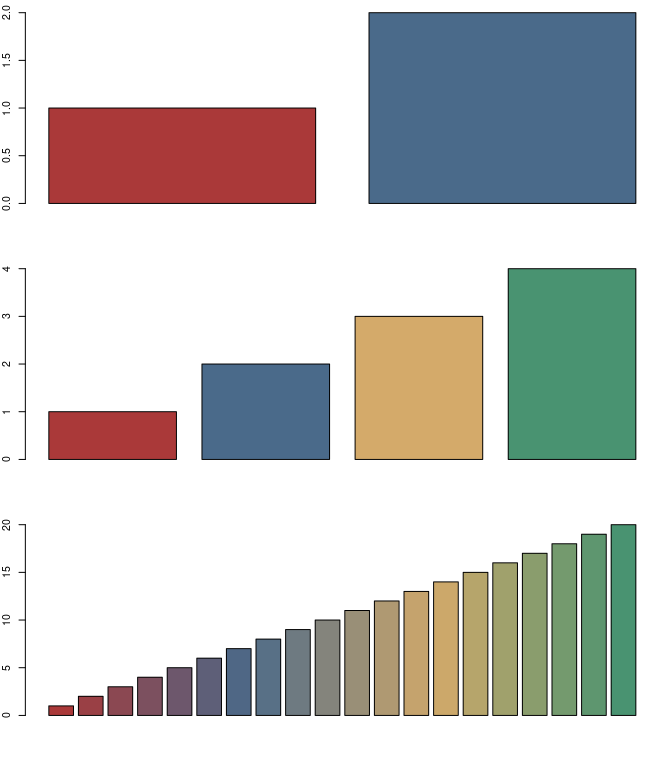This palette also has a light and a dark version:

par(mfrow = c(3,1))
barplot(1:20, col = incidence_pal1_dark(20), main = "palette:  incidence_pal1_dark")
barplot(1:20, col = incidence_pal1(20), main = "palette:  incidence_pal1")
barplot(1:20, col = incidence_pal1_light(20), main = "palette:  incidence_pal1_light")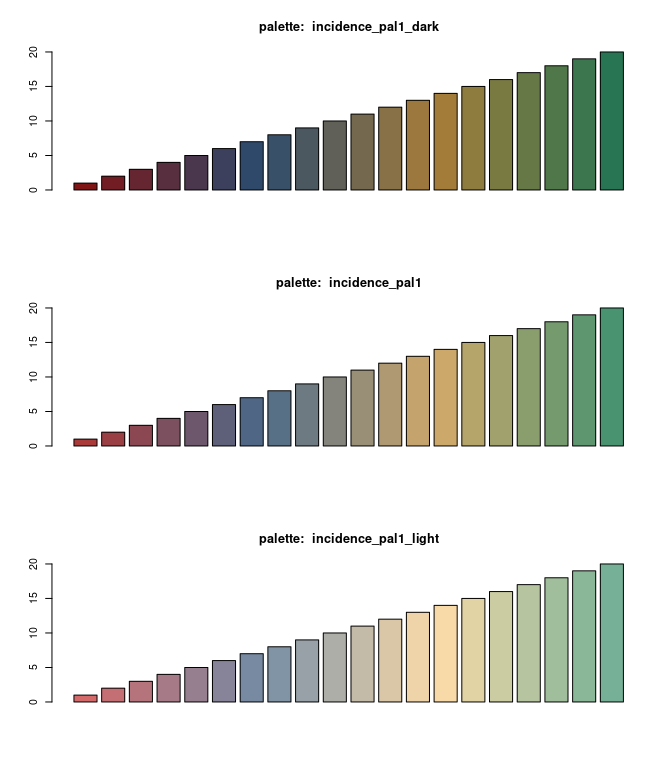### Using different palettes

Other color palettes can be provided via col_pal. Various palettes are part of the base R distribution, and many more are provided in additional packages. We provide a couple of examples:

plot(i.hosp, col_pal = rainbow)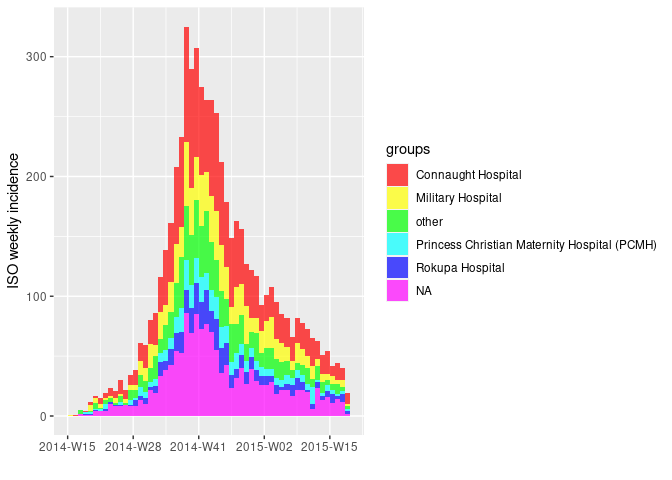plot(i.sex, col_pal = cm.colors)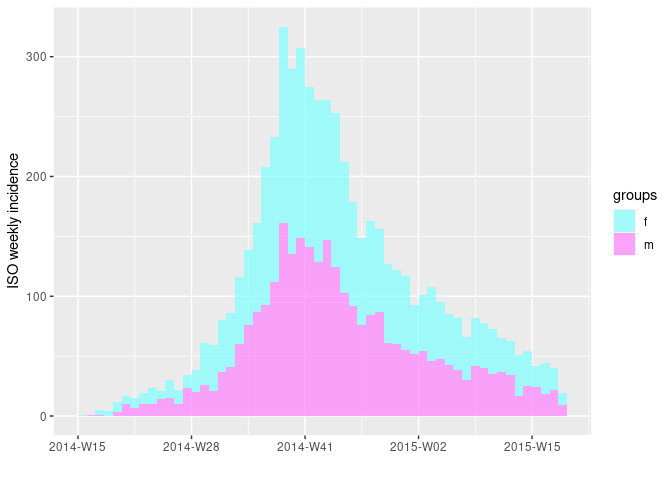### Specifying colors manually

Colors can be specified manually using the argument color; note that whenever incidence is computed by groups, the number of colors must match the number of groups, otherwise color is ignored.

#### Example 1: changing a single color

plot(i, color = "darkred")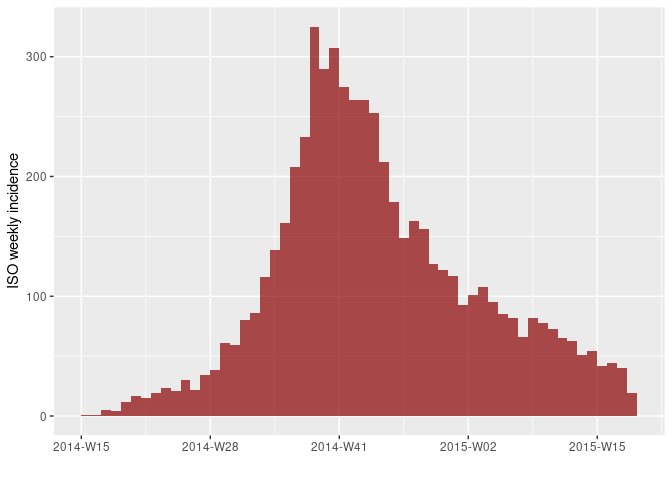#### Example 2: changing several colors (note that naming colors is optional)

plot(i.sex, color = c(m = "orange2", f = "purple3"))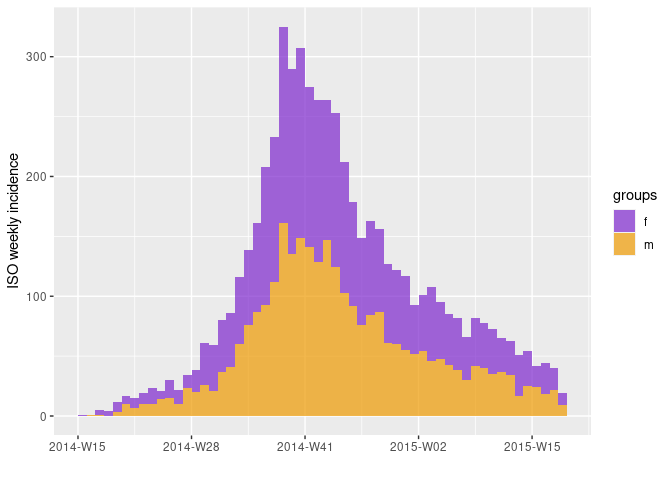#### Example 3: using color to highlight specific groups

plot(i.hosp,
color = c("#ac3973", "#6666ff", "white", "white", "white", "white"))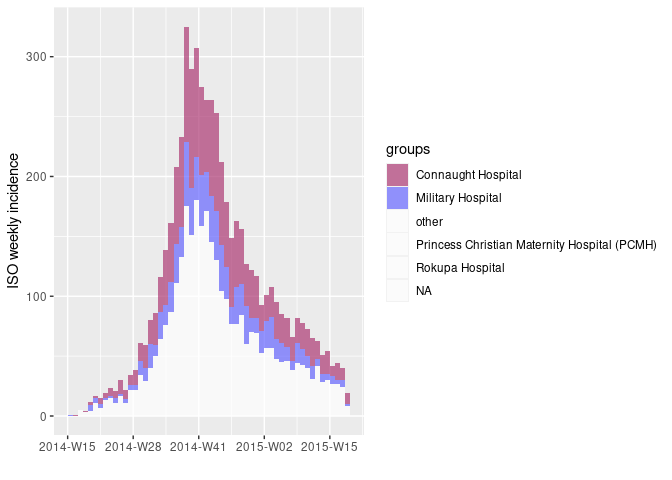# Useful ggplot2 tweaks

Numerous tweaks for ggplot2 are documented online. In the following, we merely provide a few useful tips in the context of incidence.

## Changing dates on the x-axis

### Changing date format

By default, the dates indicated on the x-axis of an incidence plot may not have the suitable format. The package scales can be used to change the way dates are labeled (see ?strptime for possible formats):

library(scales)
plot(i, labels_week = FALSE) +
scale_x_date(labels = date_format("%d %b %Y"))
#> Scale for 'x' is already present. Adding another scale for 'x', which will
#> replace the existing scale.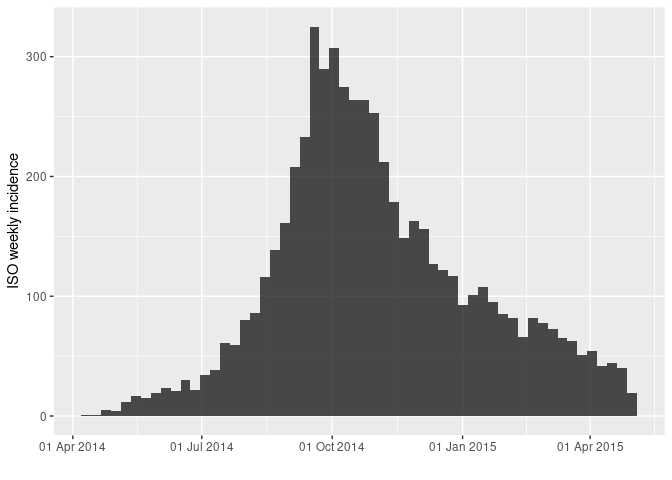Notice how the labels are all situated at the first of the month? If you want to make sure the labels are situated in a different orientation, you can use the make_breaks() function to calculate breaks for the plot:

b <- make_breaks(i, labels_week = FALSE)
b
#> $breaks #>  "2014-04-07" "2014-07-07" "2014-10-06" "2015-01-05" "2015-04-06" #>  "2015-07-06" #> #>$labels
#> list()
#> attr(,"class")
#>  "waiver"
plot(i) +
scale_x_date(breaks = b$breaks, labels = date_format("%d %b %Y")) #> Scale for 'x' is already present. Adding another scale for 'x', which will #> replace the existing scale.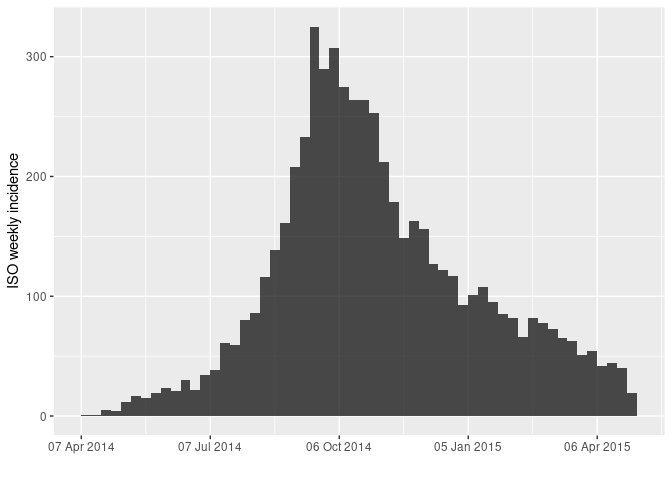And for another example, with a subset of the data (first 50 weeks), using more detailed dates and rotating the annotations: plot(i[1:50]) + scale_x_date(breaks = b$breaks, labels = date_format("%a %d %B %Y")) +
theme(axis.text.x = element_text(angle = 45, hjust = 1, size = 12))
#> Scale for 'x' is already present. Adding another scale for 'x', which will
#> replace the existing scale.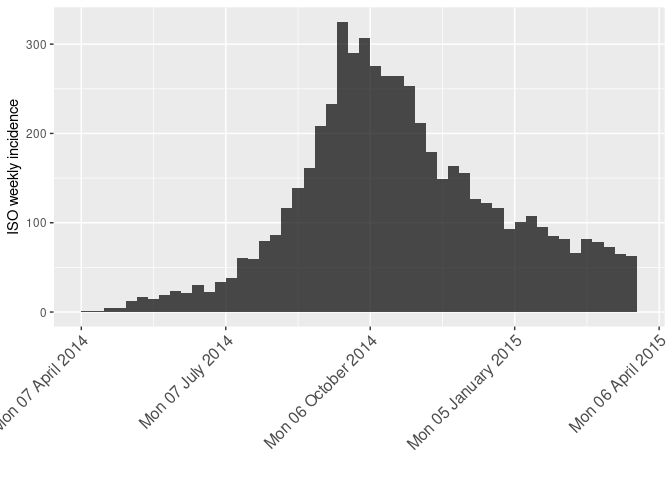Note that you can save customisations for later use:

rotate.big <- theme(axis.text.x = element_text(angle = 45, hjust = 1, size = 12))

### Changing the grid

The last example above illustrates that it can be useful to have denser annotations of the x-axis, especially over short time periods. Here, we provide an example where we try to zoom on the peak of the epidemic, using the data by hospital:

plot(i.hosp)Let us look at the data 40 days before and after the 1st of October:

period <- as.Date("2014-10-01") + c(-40, 40)
i.zoom <- subset(i.hosp, from = period, to = period)
detailed.x <- scale_x_date(labels = date_format("%a %d %B %Y"),
date_breaks = "2 weeks",
date_minor_breaks = "week")

plot(i.zoom, border = "black") + detailed.x + rotate.big
#> Scale for 'x' is already present. Adding another scale for 'x', which will
#> replace the existing scale.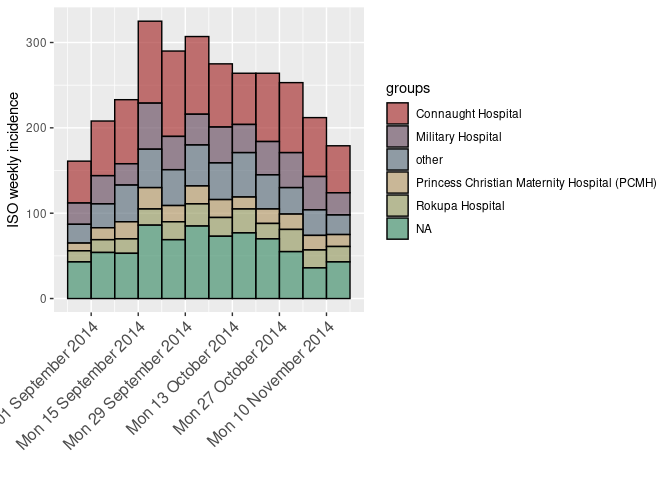### Handling non-ISO weeks

If you have weekly incidence that starts on a day other than monday, then the above solution may produce breaks that fall inside of the bins:

i.sat <- incidence(onset, interval = "1 week: saturday", groups = ebola_sim_clean$linelist$hospital)
i.szoom <- subset(i.sat, from = period, to = period)

plot(i.szoom, border = "black") + detailed.x + rotate.big
#> Scale for 'x' is already present. Adding another scale for 'x', which will
#> replace the existing scale.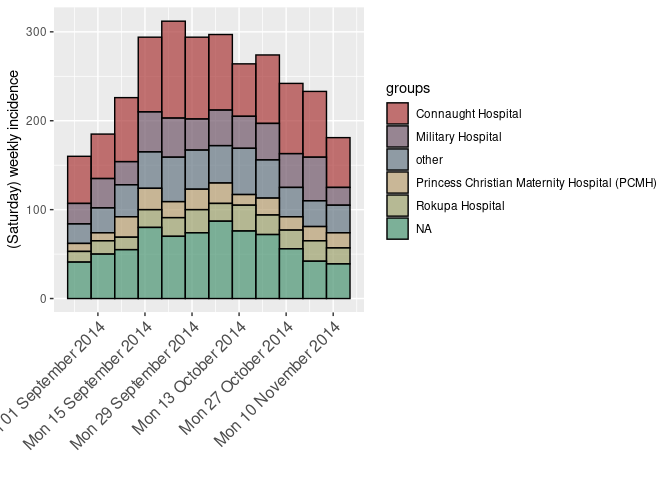In this case, you may want to either calculate breaks using make_breaks() or use the scale_x_incidence() function to automatically calculate these for you:

plot(i.szoom, border = "black") +
scale_x_incidence(i.szoom, n_breaks = nrow(i.szoom)/2, labels_week = FALSE) +
rotate.big
#> Scale for 'x' is already present. Adding another scale for 'x', which will
#> replace the existing scale.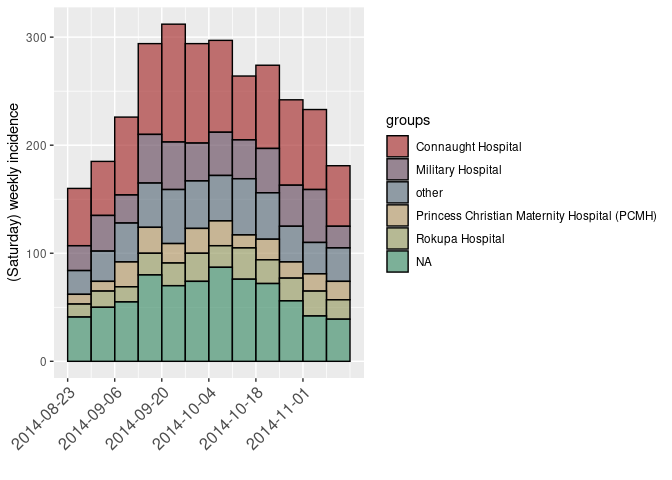sat_breaks <- make_breaks(i.szoom, n_breaks = nrow(i.szoom)/2)
plot(i.szoom, border = "black") +
scale_x_date(breaks = sat_breaks\$breaks, labels = date_format("%a %d %B %Y")) +
rotate.big
#> Scale for 'x' is already present. Adding another scale for 'x', which will
#> replace the existing scale.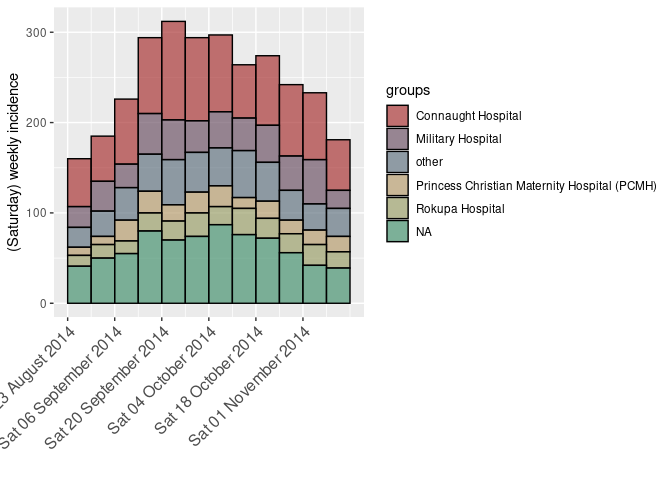### Labelling every bin

Sometimes you may want to label every bin of the incidence object. To do this, you can simply set n_breaks to the number of rows in your incidence object:

plot(i.szoom, n_breaks = nrow(i.szoom), border = "black") + rotate.big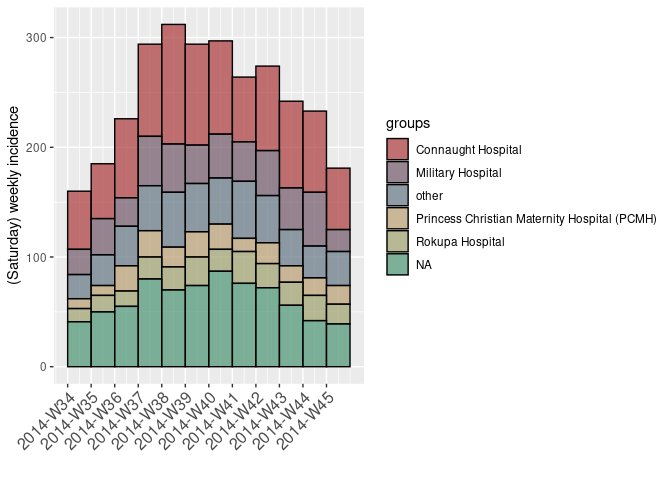## Changing the legend

The previous plot has a fairly large legend which we may want to move around. Let us save the plot as a new object p and customize the legend:

p <- plot(i.zoom, border = "black") + detailed.x + rotate.big
#> Scale for 'x' is already present. Adding another scale for 'x', which will
#> replace the existing scale.
p + theme(axis.text.x = element_text(angle = 45, hjust = 1, size = 12),
legend.position = "top", legend.direction = "horizontal",
legend.title = element_blank())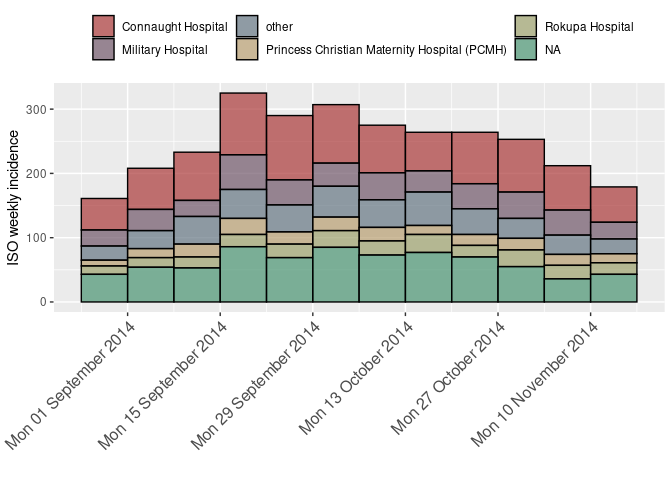## Applying the style of European Programme for Intervention Epidemiology Training (EPIET)

### Display individual cases

For small datasets it is convention of EPIET to display individual cases as rectangles. It can be done by doing two things: first, adding using the option show_cases = TRUE with a white border and second, setting the background to white. We also add coord_equal() which forces each case to be a square.

i.small <- incidence(onset[160:180])

plot(i.small, border = "white", show_cases = TRUE) +
theme(panel.background = element_rect(fill = "white")) +
rotate.big +
coord_equal()